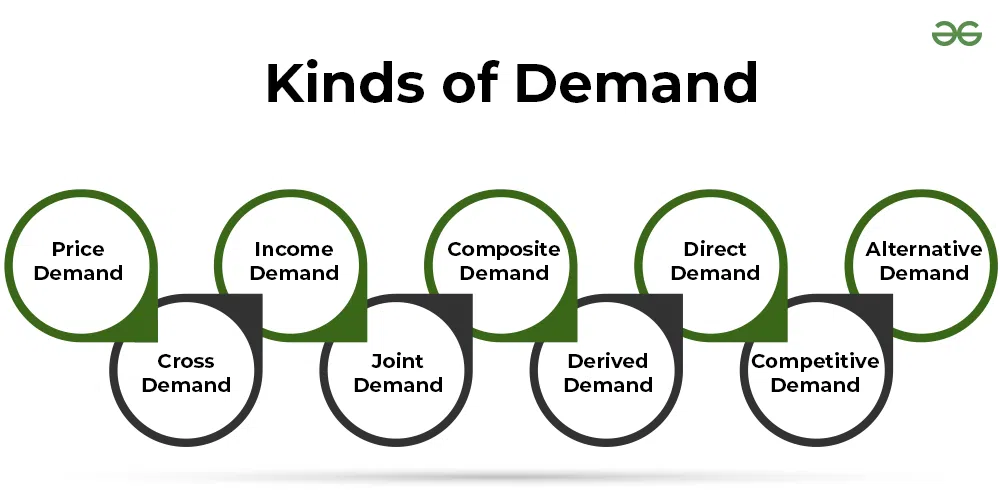GFG App
Open AppBrowser
Continue

In economics, demand is the quantity of a good or service that a consumer is willing and able to purchase at different price levels available during a given time period. Although the demand is the desire of a consumer to purchase a commodity, it is not the same as desire. Desire is just a wish of a consumer to purchase a commodity even though he is unable to buy it. However, demand is a consumer’s desire to purchase a commodity, provided he is willing to spend and has sufficient purchasing power.
Hence, we can say that the four essential elements of demand are:

• Quantity of the commodity
• Willingness of a consumer to purchase the commodity
• Time period
• Price of the commodity at each quantity level

### Types of DemandThe nine different types of demand are as follows:

#### 1. Price Demand:

Assuming other factors as constant, a relationship between the price and demand of a commodity is known as Price Demand. Price Demand can be shown as:

Dx = f(Px)

Where,

Dx = Demand for the given Commodity

f = Functional Relationship

Px = Price of the given Commodity

#### 2. Cross Demand:

Assuming other things remaining as constant, a relationship between the demand of a given commodity and the price of related commodities is known as Cross Demand.

#### 3. Income Demand:

Assuming other factors as constant, a relationship between the consumer’s income and the quantity demanded for a commodity is known as Income Demand. Income Demand can be shown as:

Dx = f(Y)

Where,

Dx = Demand for the given Commodity

f = Functional Relationship

Y = Income of the Consumer

#### 4. Joint Demand:

When demand for two or more goods arises simultaneously for satisfying a particular want of the consumer, then such type of demand is known as Joint Demand. For example, the demand for milk, coffee beans, and sugar is a joint demand as all these goods are demanded together to prepare coffee.

#### 5. Composite Demand:

When a commodity can be used for more than one purpose, then such type of demand is known as Composite Demand. For example, the demand for water is a composite demand as it can be used for various purposes like bathing, drinking, cooking, etc.

#### 6. Derived Demand:

The kind of demand for a commodity, which depends on the demand for other goods, is known as Derived Demand. For example, demand for workers/labour, producing bags is a derived demand as it depends on the demand for bags.

#### 7. Direct Demand:

When a commodity directly satisfies the demand of consumers, then its demand is known as Direct Demand. For example, demand for books, stationery, clothes, food, etc., is a direct demand as these goods directly satisfy the wants.

#### 8. Competitive Demand:

When two commodities are close substitutes of each other and an increase in the demand for one commodity will decrease the demand for the other commodity, then the demand for any one of the commodities is known as Competitive Demand. For example, an increase in demand for tea might decrease the demand for coffee, which makes the demand for these goods competitive demand. This happens because when consumers purchase more of one commodity (say tea), it leads to a lesser requirement for the other commodity (say coffee).

#### 9. Alternative Demand:

Demand for a commodity is known as alternative demand when it can be satisfied by using different alternatives. For example, there are number of alternatives to satisfy the demand for clothes like jeans, shirts, trousers, suits, saree, pants, etc.

My Personal Notes arrow_drop_up